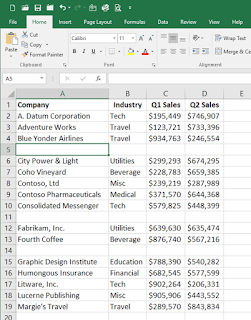Friday, January 12, 2018

How to Calculate Percentages in Excel

Did you get your annual raise yet?

Many people have asked me over the years, "How do I calculate my annual raise when I know what percentage I'm getting?"

First, let's understand percentages. The term "percent" is literally broken down as "per," which means "out of" and "cent," which is one-hundred. So, a percent is the number out of one-hundred.

So, you're probably asking, "Debbie, how does that help me when I was told I was getting a 3% salary increase?"

Well, here's how you would calculate it.

Let's take Jim. Jim was told by his boss that he would be receiving a 3% salary increase on January 15th. If Jim's current salary is \$40,000, let's see what his raise would be.

If you'd like to use Excel to do this, simply write the formula as such.

Column A would contain Jim's current salary of \$40,000.

Column B would contain Jim's increase percentage of 3%.

Column C would calculate the 3% of \$40,000, which would output \$1,2000.

Column D would then add the \$1,200 increase to Jim's current salary of \$40,000, which would show his NEW Salary of \$41,200.

Now, this is a bit of the long way around, but if it works for you, go ahead and use it.

For those of you who like quicker ways to calculate increases, here's what you would do. (Example shown in screenshot, Row 5)

Column A would contain Jim's current salary of \$40,000.

Column B would contain the formula =A5*1.03 (this means you are saying I want 100% of the current salary, PLUS 3% added). This then gives you the \$41,200 NEW Salary figure we came up with the 4 Column process above.

Hopefully, many of you will receive more than 3% with the new U.S. Tax Reform announced recently! But, this is just an example of how you can easily calculate percentages using Excel.